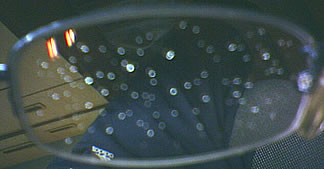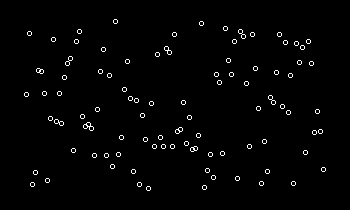# Random Number Generation on the Glasses (A Natural RNG)

### Yihui Xie 2008-05-11

I was unfortunately caught in the rain this evening and my glasses were blurred by the rain drops. When I came back the office, took them off and was about to clear the beads, I just found a good RNG (Random Number Generator) from the nature:People who are familiar with statistical computation must have learned how to generate random numbers following a `U(0, 1)` distribution. One of the most common generators is the linear congruential generator. This is just what I was reminded of by the rain drops on my glasses. The plot below is made in R based the linear congruential generator:

``````n = 100
x = numeric(n + 1)
# the seed: generated from the system clock (number of seconds from 00:00)
x = sum(as.integer(substring(Sys.time(), c(12, 15, 18),
c(13, 16, 19))) * c(3600, 60, 1))/(24 * 60 * 60)
for (i in 1:n) x[i + 1] = (7^5 * x[i])%%(2^31 - 1)
# "normalize" to [0, 1]
x = x/(2^31 - 1)
op = par(mar = c(1, 1, 1, 1), bg = "black")
plot(x, col = "white")
par(op)
``````Do they look like each other? Here is a simple animation.